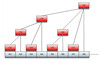log（n）

• 树状数组
2021-02-27 20:02:03

先来看几个问题吧。

1.什么是树状数组？

顾名思义，就是用数组来模拟树形结构呗。那么衍生出一个问题，为什么不直接建树？答案是没必要，因为树状数组能处理的问题就没必要建树。和Trie树的构造方式有类似之处。

2.树状数组可以解决什么问题

可以解决大部分基于区间上的更新以及求和问题。

3.树状数组和线段树的区别在哪里

树状数组可以解决的问题都可以用线段树解决，这两者的区别在哪里呢？树状数组的系数要少很多，就比如字符串模拟大数可以解决大数问题，也可以解决1+1的问题，但没人会在1+1的问题上用大数模拟。

4.树状数组的优点和缺点

修改和查询的复杂度都是O(logN)，而且相比线段树系数要少很多，比传统数组要快，而且容易写。

缺点是遇到复杂的区间问题还是不能解决，功能还是有限。

一、树状数组介绍

二叉树大家一定都知道，如下图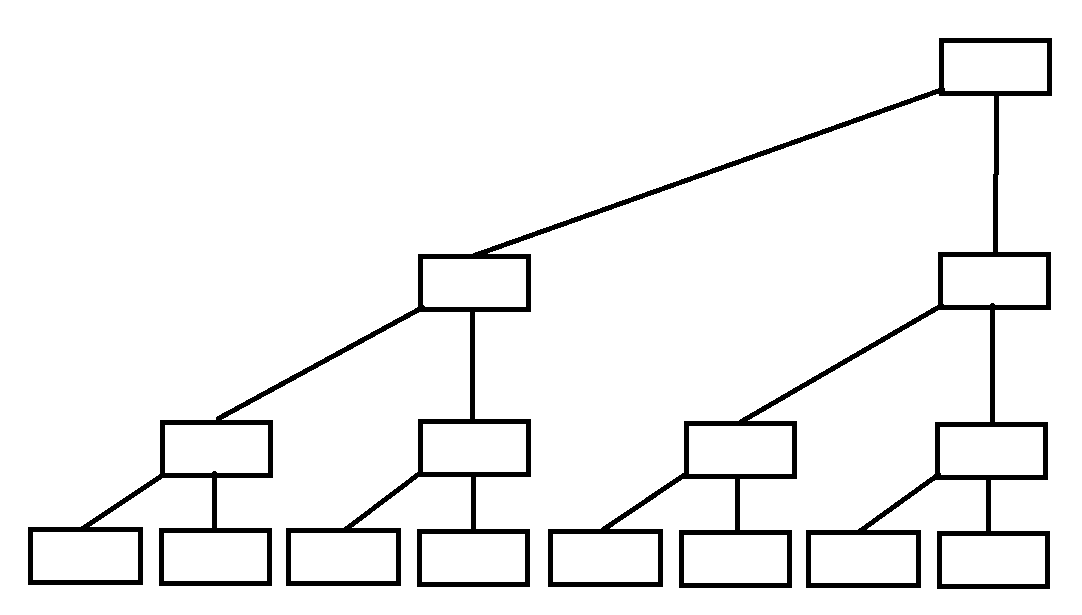如果每个父亲都存的是两个儿子的值，是不是就可以解决这类区间问题了呢。是的没错，但是这样的树形结构，叫做线段树。

那真的的树形结构是怎样的，和上图类似，但省去了一些节点，以达到用数组建树。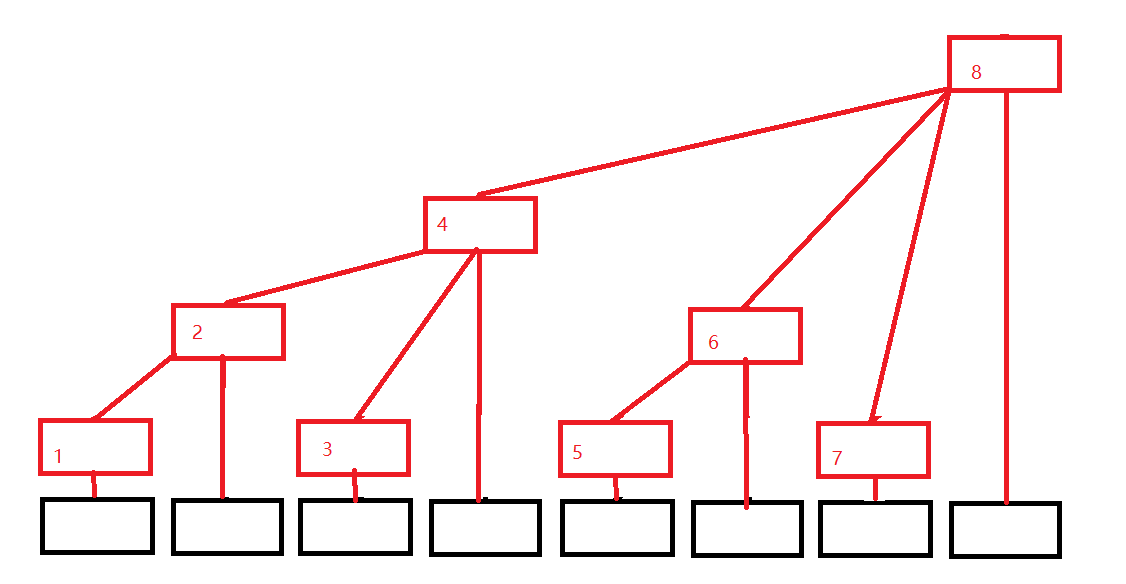黑色数组代表原来的数组(下面用A[i]代替)，红色结构代表我们的树状数组(下面用C[i]代替)，发现没有，每个位置只有一个方框，令每个位置存的就是子节点的值的和，则有

C = A;

C = A + A;

C = A;

C = A + A + A + A;

C = A;

C = A + A;

C = A;

C = A + A + A + A + A + A + A + A;

可以发现，这颗树是有规律的

C[i] = A[i - 2k+1] + A[i - 2k+2] + ... + A[i];   //k为i的二进制中从最低位到高位连续零的长度

例如i = 8(1000)时候，k = 3，可自行验证。

这个怎么实现求和呢，比如我们要找前7项和，那么应该是SUM = C + C + C;

而根据上面的式子，容易的出SUMi = C[i] + C[i-2k1] + C[(i - 2k1) - 2k2] + .....；

其实树状数组就是一个二进制上面的应用。

现在新的问题来了2^k该怎么求呢，不难得出2^k = i&(i^(i-1));但这个还是不好求出呀，前辈的智慧就出来了，2^k = i&(-i);

为什么呢？

这里利用的负数的存储特性，负数是以补码存储的，对于整数运算 x&(-x)有

● 当x为0时，即 0 & 0，结果为0；

●当x为奇数时，最后一个比特位为1，取反加1没有进位，故x和-x除最后一位外前面的位正好相反，按位与结果为0。结果为1。

●当x为偶数，且为2的m次方时，x的二进制表示中只有一位是1(从右往左的第m+1位)，其右边有m位0，故x取反加1后，从右到左第有m个0，第m+1位及其左边全是1。这样，x& (-x) 得到的就是x。

●当x为偶数，却不为2的m次方的形式时，可以写作x= y * (2^k)。其中，y的最低位为1。实际上就是把x用一个奇数左移k位来表示。这时，x的二进制表示最右边有k个0，从右往左第k+1位为1。当对x取反时，最右边的k位0变成1，第k+1位变为0；再加1，最右边的k位就又变成了0，第k+1位因为进位的关系变成了1。左边的位因为没有进位，正好和x原来对应的位上的值相反。二者按位与，得到：第k+1位上为1，左边右边都为0。结果为2^k。

总结一下：x&(-x)，当x为0时结果为0；x为奇数时，结果为1；x为偶数时，结果为x中2的最大次方的因子。

而且这个有一个专门的称呼，叫做lowbit，即取2^k。

二、如何建立树状数组

上面已经解释了如何用树状数组求区间和，那么如果我们要更新某一个点的值呢，还是一样的，上面说了C[i] = A[i - 2k+1] + A[i - 2k+2] + ... + A[i]，那么如果我们更新某个A[i]的值，则会影响到所有包含有A[i]位置。如果求A[i]包含哪些位置里呢，同理有

A[i] 包含于 C[i + 2k]、C[(i + 2k) + 2k]...；

好，现在已经搞清楚了更新和求和，就可以来建树状数组了。如果上面的求和、更新或者lowbit步骤还没搞懂的化，建议再思考弄懂再往下看。

那么构造一个树状数组则为

1 intn;2 int a,c; //对应原数组和树状数组

3

4 int lowbit(intx){5 return x&(-x);6 }7

8 void updata(int i,int k){ //在i位置加上k

9 while(i <=n){10 c[i] +=k;11 i +=lowbit(i);12 }13 }14

15 int getsum(int i){ //求A[1 - i]的和

16 int res = 0;17 while(i > 0){18 res +=c[i];19 i -=lowbit(i);20 }21 returnres;22 }

这样就构造了一个树状数组。下面看一道模板题目吧。

直接看代码吧

1 #include

2 using namespacestd;3

4 intn,m;5 int a,c; //对应原数组和树状数组

6

7 int lowbit(intx){8 return x&(-x);9 }10

11 void updata(int i,int k){ //在i位置加上k

12 while(i <=n){13 c[i] +=k;14 i +=lowbit(i);15 }16 }17

18 int getsum(int i){ //求A[1 - i]的和

19 int res = 0;20 while(i > 0){21 res +=c[i];22 i -=lowbit(i);23 }24 returnres;25 }26

27 intmain(){28 intt;29 cin>>t;30 for(int tot = 1; tot <= t; tot++){31 cout << "Case" << tot << ":" <>n;35 for(int i = 1; i <= n; i++){36 cin>>a[i];37 updata(i,a[i]); //输入初值的时候，也相当于更新了值

38 }39

40 strings;41 intx,y;42 while(cin>>s && s != 'E'){43 cin>>x>>y;44 if(s == 'Q'){ //求和操作

45 int sum = getsum(y) - getsum(x-1); //x-y区间和也就等于1-y区间和减去1-(x-1)区间和

46 cout << sum <

53 }54 }55

56 }57 return 0;58 }

这就是最简单的点更新区间求和了。

三、树状数组的几种变式(区间更新，区间查询)

上面介绍的是最普通的单点更新，区间查询，但如果有些时候是区间更新，单点求和怎么半，又或是区间更新，区间求和怎么办。这里将介绍各种情况该怎么写。

如果上面的单点更新，区间查询还没看懂，建议再思考再往下看。

1.单点更新、单点查询

传统数组可做

2.单点更新、区间查询

已讲解，详细看上面

3.区间更新、单点查询

这就是第一个问题，如果题目是让你把x-y区间内的所有值全部加上k或者减去k，然后查询操作是问某个点的值，这种时候该怎么做呢。如果是像上面的树状数组来说，就必须把x-y区间内每个值都更新，这样的复杂度肯定是不行的，这个时候，就不能再用数据的值建树了，这里我们引入差分，利用差分建树。

假设我们规定A = 0;

则有 A[i] = Σij = 1D[j];(D[j] = A[j] - A[j-1])，即前面i项的差值和，这个有什么用呢？例如对于下面这个数组

A[] = 1 2 3 5 6 9

D[] = 1 1 1 2 1 3

如果我们把[2,5]区间内值加上2，则变成了

A[] = 1 4 5 7 8 9

D[] = 1 3 1 2 1 1

发现了没有，当某个区间[x,y]值改变了，区间内的差值是不变的，只有D[x]和D[y+1]的值发生改变，至于为什么我想我就不用解释了吧。

所以我们就可以利用这个性质对D[]数组建立树状数组，代码为：

1 intn,m;2 int a = {0},c; //对应原数组和树状数组

3

4 int lowbit(intx){5 return x&(-x);6 }7

8 void updata(int i,int k){ //在i位置加上k

9 while(i <=n){10 c[i] +=k;11 i +=lowbit(i);12 }13 }14

15 int getsum(int i){ //求D[1 - i]的和，即A[i]值

16 int res = 0;17 while(i > 0){18 res +=c[i];19 i -=lowbit(i);20 }21 returnres;22 }23

24 intmain(){25 cin>>n;27 for(int i = 1; i <= n; i++){28 cin>>a[i];29 updata(i,a[i] - a[i-1]); //输入初值的时候，也相当于更新了值

31 }32

33 //[x,y]区间内加上k

34 updata(x,k); //A[x] - A[x-1]增加k

35 updata(y+1,-k); //A[y+1] - A[y]减少k36

37 //查询i位置的值

38 int sum =getsum(i);39

40 return 0;41 }

这样就把，原来要更新一个区间的值变成了只需要更新两个点。也很容易理解吧。

4.区间更新、区间查询

上面我们说的差值建树状数组，得到的是某个点的值，那如果我既要区间更新，又要区间查询怎么办。这里我们还是利用差分，由上面可知

∑ni = 1A[i] = ∑ni = 1 ∑ij = 1D[j];

则A+A+...+A[n]

= (D) + (D+D) + ... + (D+D+...+D[n])

= n*D + (n-1)*D +... +D[n]

= n * (D+D+...+D[n]) - (0*D+1*D+...+(n-1)*D[n])

所以上式可以变为∑ni = 1A[i] = n*∑ni = 1D[i] -  ∑ni = 1( D[i]*(i-1) );

如果你理解前面的都比较轻松的话，这里也就知道要干嘛了，维护两个数状数组，sum1[i] = D[i]，sum2[i] = D[i]*(i-1);

1 intn,m;2 int a = {0};3 int sum1; //(D + D + ... + D[n])

4 int sum2; //(1*D + 2*D + ... + n*D[n])

5

6 int lowbit(intx){7 return x&(-x);8 }9

10 void updata(int i,intk){11 int x = i; //因为x不变，所以得先保存i值

12 while(i <=n){13 sum1[i] +=k;14 sum2[i] += k * (x-1);15 i +=lowbit(i);16 }17 }18

19 int getsum(int i){ //求前缀和

20 int res = 0, x =i;21 while(i > 0){22 res += x * sum1[i] -sum2[i];23 i -=lowbit(i);24 }25 returnres;26 }27

28 intmain(){29 cin>>n;30 for(int i = 1; i <= n; i++){31 cin>>a[i];32 updata(i,a[i] - a[i-1]); //输入初值的时候，也相当于更新了值

33 }34

35 //[x,y]区间内加上k

36 updata(x,k); //A[x] - A[x-1]增加k

37 updata(y+1,-k); //A[y+1] - A[y]减少k38

39 //求[x,y]区间和

40 int sum = getsum(y) - getsum(x-1);41

42 return 0;43 }

再附赠两道模板题目，可以自行写一下以便理解

区间修改、单点查询模板题目：https://www.luogu.org/problem/show?pid=3368

区间修改、区间查询模板题目：https://vjudge.net/problem/POJ-3468

PS：这里大致归纳了一维树状数组的所有要使用到的东西，二维建树以及更多变式就不说了，具体问题再具体分析。

后记：自己看了一下写的不是很好，特别是公式和图，都是用简单的画图和直接写的，没有用编辑器，也不能说我懒吧，毕竟精力有限啦，以后有空还是会去学的，带给大家更好的博客。手敲也不易，希望大家理解，多多支持。

不懂问我噢= =

更多相关内容
• 树状数组简单来说就是仅通过一个数组来描述一棵或一个森林。数组中每个元素只存储着中对应节点存储的信息，不存储描述的层次结构的信息。 2.普通的存储 对于一棵普通的，在《数据结构》严蔚敏版中提到可以...

# 1.树状数组概念

树状数组简单来说就是仅通过一个数组来描述一棵树或一个森林。数组中每个元素只存储着树中对应节点存储的信息，不存储描述树的层次结构的信息。

# 2.普通树的存储

对于一棵普通的树，在《数据结构》严蔚敏版中提到可以使用如下方法进行存储：

### 2.1 父节点表示法

用一个数组中存储节点信息，节点信息包括节点存储数据和父节点编号。

struct Node {
int data; // 节点存储的数据
int parent; // 节点对应的父节点的编号
}

struct Tree {
struct Node* node; // 存储节点的数组的指针
int r; // 树中根节点的编号
int n; // 树中节点个数
}


### 2.2 孩子表示法

用一个数组存储节点信息，节点信息包括节点存储数据和子节点链表。

struct Child {
int data; // 节点存储的数据
int childNumber; // 该节点是第几个孩子
struct Child* nextChild; // 指向下一个孩子的指针
}

struct Node {
int data; // 该节点存储的信息
struct Child* firstChild; // 该节点的孩子数组的指针
}

struct Tree {
struct Node* node; // 存储节点的数组的指针
int r; // 树中根节点的编号
int n; // 树中节点个数
}


### 2.3 孩子兄弟表示法

用一个数组存储节点信息，节点信息包括节点存储数据，节点下一个兄弟节点，节点第一个子节点。

想要访问某一个节点的子节点只需要从第一个子节点出发通过兄弟节点依次寻找即可。

孩子兄弟表示法也是二叉树表示法，因为每个节点有两个指针。

struct Node {
int data; // 节点存储的数据
struct Node* nextSibiling; // 指向下一个兄弟节点的指针
struct Node* firstChild; // 指向第一个子节点的指针
}

struct Tree {
struct Node* node; // 存储节点的数组的指针
int r; // 树中根节点的编号
int n; // 树中节点个数
}


### 2.4 遍历序列表示法

树的先序+后序遍历数组不能唯一确定一棵树。

树的先序+中序遍历数组能唯一确定一棵树。

树的后序+中序遍历数组能唯一确定一棵树。

这种表示方法是使用两个数组来存储节点信息，节点信息只包括节点存储数据，不包含对树层次结构的描述。

# 3.树状数组树的存储

在1中提到树状数组只是一个数组且不存储树或森林的层次结构信息，这和2中的几种方法对比即可发现，这是不可能准确描述一棵树的。实际上树状数组拥有特殊性质，层次结构信息在树状数组中不需要额外描述。注：树状数组描述的不一定是一个树，也有可能是一个森林，下文为了表述简便期间，用树状数组树代替可能产生的树或森林。

### 3.1 树状数组和树状数组树的核心性质

1. (数组下标和树中节点编号对应) 树状数组中的每个元素的下标都对应着树状数组树中的节点的编号，树状数组中第一个元素就是树状数组树中编号为1的节点。
2. (树状数组第一个元素对应树中节点) 树状数组中第一个元素是树状数组树的第一个叶子结点。
3. (树状数组树中节点的层次关系) 树状数组树中的某个节点编号为i，它的父节点编号是i + (i & (-i))。由此可向上追溯到祖先节点。树状数组树中的某个节点编号为i，编号小于i且层级最高的节点编号是是i - (i & (-i))

### 3.2 由树状数组创建一课树状数组树

给出一个树状数组ar，由此创建一棵树状数组树。

ar = [x1, x2, x3, x4, x5, x6, x7, x8]x表示对应元素存储的信息，我们在此用x替代，我们在3.2只关注如何创建树状数组树。

**特别注意：下面的图中指明了ar数组的下标从1开始，在程序中存储时也应注意，如果从0开始存储，在计算第一个节点的父节点，也就是i + (i & (-i))时会始终计算为0，原因是0的补码导致，在此不是终点，可以查阅计算机组成原理相关知识。 **

第一步： 从第一个元素开始遍历树状数组

根据3.1性质1和2可知，树状数组的第一个元素对应着树状数组树中的第一个叶子结点，并且该节点编号为1。根据3.1性质3可知，树状数组树的层次结构中的父节点编号可以由当前节点编号推出。经过计算，可以一直推导出祖先节点。

8号节点当然可以继续往后推导，但是会超过树状数组的界限，树状数组只存储了8个树中节点的信息。第二步： 遍历到第二个元素

树状数组中第二个元素对应的下标2已经存在在步骤一构造的树中，这代表着树状数组中下标为2的元素对应的树中的节点2已经被创建，所以直接跳过即可。

第三步： 遍历到第三个元素

第三个元素对应的节点还未被创建，因为上图中的节点还没有编号为3。因此使用同样的规则创建节点。第四步： 遍历到第四个元素

4号节点已被创建，跳过

第五步： 遍历到第五个元素第六步： 遍历到第六个元素

6号节点已在树中存在，跳过

第七步： 遍历到第七个元素第八步： 遍历到第八个元素

8号节点已在树中存在，跳过。

注：如果树状数组仅有7个元素，那么就是把上图的8号节点去掉，这时产生的就是一个森林。

# 4.树状数组的基本功能

1. update函数表示在树状数组树中更新某个节点的值，并以O(logn)时间复杂度沿着该节点向上到祖先节点，更新这条路径上经过的节点的值。

2. getSum函数表示在树状数组中以O(logn)时间复杂度求前缀和。

3. 树状数组的核心在于树状数组树的叶子结点存储什么样的信息，叶子节点存储的信息在使用时会通过getSum以前缀和形式使用。

只需知道树状数组的功能，工作原理会在后文介绍。

int lowbit(int x) {
return x & (-x);
}

void update(int *ar, int pos, int n, int val) {
while(pos <= n) {
ar[pos] += val;
pos += lowbit(pos);
}
}

int getSum(int *ar, int pos) {
int sum = 0;
while(pos >= 1) {
sum += ar[pos];
pos -= lowbit(pos);
}
return sum;
}


# 5.树状数组数据结构的优势

从3中的过程可知树状数组的建立的时间复杂度为O(n)。

### 5.1 单点更新&区间查询

背景：

原数列中进行多轮修改操作，每轮修改操作会修改其中某一个数的值，每轮修改后询问一个特定区间的和

树状数组(树)存储信息：

树状数组树的叶子结点存储的值和原数列对应位置的值相同。

优势：

O(logn)区间更新 O(logn)单点查询

#### 5.1.1 修改树状数组的创建

在这种情况下给出树状数组树的创建（也可以说是树状数组的创建）的代码。

已知一个序列，要求创建出序列对应的树状数组ar

#include <iostream>
using namespace std;

int lowbit(int x) {
return x & (-x);
}

void update(int *ar, int pos, int n, int val) {
while(pos <= n) {
ar[pos] += val;
pos += lowbit(pos);
}
}

int main() {
int n, x;
cin >> n;
int ar[n + 1];
for(int i = 1; i <= n; i ++)
ar[i] = 0;
for(int i = 1; i <= n; i ++) {
cin >> x;
update(ar, i, n, x);
}

for(int i = 1; i <= n; i ++)
cout << ar[i] << " ";
}

// 测试数据
// 8
// 1 2 3 4 5 6 7 8
// 结果
// 1 3 3 10 5 11 7 36


#### 5.1.2 区间和查询

因为树状数组树的每个节点的元素都存储着其所有子节点存储值的和，那么原数列中前i个元素的前缀和就可以由树状数组树的第i个节点以及第i个节点之前的所有层级最高的节点所存储值的和来表示。利用了3.1中的性质3int getSum(int *ar, int pos) {
int sum = 0;
while(pos >= 1) {
sum += ar[pos];
pos -= lowbit(pos); // 找到编号小于pos，且层级最高的那个节点
}
return sum;
}


由此可以获得前缀和，时间复杂度是O(logn)。由前缀和可以求得区间和。getSum(ar, 5) - getSum(ar, 1)可以获得[2, 5]区间的区间和。

#### 5.1.3 单点更新

单点更新直接调用update函数即可。

### 5.2 区间更新&单点查询

背景：

原数列中进行多轮修改操作，每轮修改操作会修改其中某一个区间的所有值，给区间中每个数都加上或减去一个新的数。每轮修改后都会查询序列中某个点的值。

树状数组(树)存储信息：

树状数组树的叶子结点存储的值和原数列的差分数列对应位置的值。

优势：

O(logn)区间更新 O(logn)单点查询

#### 5.2.1 修改树状数组的创建

如果是使用4.2.1中的树状数组创建方式，即在创建时每次树状数组更新函数接收序列值a[i]。这样在区间更新时需要对每个树状数组元素进行更新操作，与直接在原序列上更新无异。此时可以使用差分的方法创建树状数组。（差分可以参考另一篇博客“前缀和与差分”）

#include <iostream>
using namespace std;

int lowbit(int x) {
return x & (-x);
}

void update(int *ar, int pos, int n, int val) {
while(pos <= n) {
ar[pos] += val;
pos += lowbit(pos);
}
}

int getSum(int *ar, int pos) {
int sum = 0;
while(pos >= 1) {
sum += ar[pos];
pos -= lowbit(pos);
}
return sum;
}

int main() {
int n, x, y;
cin >> n;
int ar[n + 1];
for(int i = 1; i <= n; i ++)
ar[i] = 0;
for(int i = 1; i <= n; i ++) {
y = x;
cin >> x;
update(ar, i, n, i == 1 ? x : x - y);
}

for(int i = 1; i <= n; i ++)
cout << getSum(ar, i) << " ";
}

// 测试数据
// 8
// 1 2 3 4 5 6 7 8
// 结果
// 1 2 3 4 5 6 7 8


#### 5.2.2 区间修改

区间修改利用差分的特性即可。

对于原数列区间[l, r]的修改，例如对原数列区间[l, r]上的每一个数都加上k。那么原数列的差分数列b只有b[l]和b[r + 1]的值会发生变化。因为在5.2的背景下，树状数组是基于原数列的差分数列构建的，因此差分数列改变时树状数组应该修改。调用update(ar, l, n, k)update(ar, r + 1, n, -k)即可。

此时的区间修改对于差分数列是O(1)操作，对于树状数组是O(logn)操作。

#### 5.2.3 单点查询

单点查询由4.1.2可知getSum(ar, i)即可获得差分数列的第i个前缀和，即为原数列下标i的元素的值，这个操作是O(logn)的。

其实使用数列差分也可以解决“区间修改，单点查询”问题，但是数列差分在单点查询时需要求前缀和，这个操作是O(n)的。

树状数组是对数列差分解决该问题的优化。

### 5.3 区间更新&区间查询

背景：

原数列中进行多轮修改操作，每轮修改操作会修改其中某一个区间的所有值，给区间中每个数都加上或减去一个新的数。每轮修改后都会查询序列中某个区间的值的和

树状数组(树)存储信息：

一个树状数组树的叶子结点存储的值和原数列的差分数列对应位置的值。

另一树状数组的叶子结点存储的值和原数列的差分数列对应位置的值乘上该位置减一对应。

优势：

O(logn)区间更新 O(logn)单点查询

#### 5.3.1 使用差分数列记录区间更新

差分数列的主要用途就是用于区间更新后的单点查询。但是这种情况是区间更新后的区间和查询，如果只使用差分数列或者是5.2中提到的树状数组优化的差分数列，那么在计算区间和时最好是O(n)时间复杂度。由于在4中提到了树状数组的优势是求区间和，因此考虑在5.2的基础是否能构造一个特殊树状数组能够使其前缀和满足差分数列推导出的原数列前缀和，这样就可以O(logn)时间复杂度查询前缀和。

#### 5.3.1 使用差分数列推导原数列前缀和

对于原数列a，可知其差分数列b，可知其前缀和数列sum。

其中前缀和数列sum中的每一项和差分数列b中的每一项的关系如下：
s u m i = ∑ j = 1 i ( i − j + 1 ) b j s u m i = i × b 1 + ( i − 1 ) × b 2 + . . . + 2 × b i − 1 + 1 × b i sum_i=\sum^{i}_{j=1}(i-j+1)b_j\\sum_i=i\times{b_1}+(i-1)\times{b_2}+{...}+2\times{b_{i-1}}+1\times{b_i}

#### 5.3.2 尝试创建一个前缀和满足差分数列推导公式的树状数组

在4中提到树状数组的核心在于O(logn)求前缀和与树状数组树的叶子结点存储的信息。在了解差分的前提下，可知上述表达式。在了解5.2和5.1的例子后更是发现树状数组树的叶子结点存储信息不同导致前缀和结果不同，那么在此考虑是否可以令树状数组树的叶子结点存储某种特殊信息能够使调用getSum函数获取前缀和时直接得到上述结果。

#### 5.3.3 一定不存在一个树状数组的前缀和满足差分数列的推导公式

在5.1.2中提到树状数组树求前缀和，在求前缀和过程中一定包括第一个叶子结点的值，因为第一个叶子结点的值也是构建树状数组树的数列中的值。但是在上面的推导公式中可见，当i取不同值时，前缀和的计算公式中b1一定要乘上i，这就导致树状数组求前缀和一定不满足上述公式。树状数组的前缀和满足大致下列形式：
t r e e S u m i = a i + a i − l o w b i t ( i ) + . . . treeSum_i=a_i+a_{i-lowbit(i)}+{...}

#### 5.3.4 改写差分数列推导原数列前缀和公式

s u m i = ( i × b 1 + i × b 2 + . . . + i × b i − 1 + i × b i ) − ( 0 × b 1 + 1 × b 2 + . . . + ( i − 2 ) × b i − 1 + ( i − 1 ) × b i ) sum_i=(i\times{b_1}+i\times{b_2}+{...}+i\times{b_{i-1}}+i\times{b_i})-(0\times{b_1}+1\times{b_2}+{...}+(i-2)\times{b_{i-1}}+(i-1)\times{b_i})

改写该公式的目的是使得该公式尽可能与5.3.3中提到的树状数组前缀和形式接近。可以看到该公式的后半部分与树状数组的前缀和公式匹配，虽然前面有一个系数，但是该系数与下标有关，并不是不可预测的。可以看到该公式的前半部分也是与树状数组的前缀和匹配公式匹配，只是前面有一个系数i。那么这样原数列的前缀和公式可以由两个树状数组的前缀和来表示：
s u m i = i ∗ g e t S u m ( t r e e 1 , n , i ) − g e t S u m ( t r e e 2 , n , i ) sum_i=i*getSum(tree1,n,i)-getSum(tree2,n,i)
第一个树状数组的每个叶子结点i存储的是原数列的差分数列的对应值b[i]

第二个树状数组的每个叶子结点i存储的是原数列的差分数列的对应值b[i]乘上i-1

至此可知，在区间更新时的时间复杂度是O(logn)，在区间查询时的时间复杂度也是O(logn)。

# 6.树状数组序列操作例题

### 6.1 区间修改&区间查询

A Simple Problem with Integers

http://poj.org/problem?id=3468

#include <iostream>
using namespace std;
long long ar;
long long br;

long long lowbit(long long x) {
return x & (-x);
}

void update(long long* ar, int n, int pos, long long x) {
while(pos <= n) {
ar[pos] += x;
pos += lowbit(pos);
}
}

long long getSum(long long* ar, int pos) {
long long sum = 0;
while(pos >= 1) {
sum += ar[pos];
pos -= lowbit(pos);
}
return sum;
}

int main() {
int n, p, l, r;
char op;
long long x, y, extraData;
cin >> n >> p;
for(int i = 1; i <= n; i ++) {
y = x;
cin >> x;
update(ar, n, i, i == 1 ? x : x - y);
update(br, n, i, i == 1 ? 0 : (x - y) * (i - 1));
}

for(int i = 0; i < p; i ++) {
cin >> op;
if(op == 'Q') {
cin >> l >> r;
cout << getSum(ar, r) * r - getSum(br, r) - getSum(ar, l - 1) * (l - 1) + getSum(br, l - 1) << endl;
} else {
cin >> l >> r >> extraData;
update(ar, n, l, extraData);
update(br, n, l, extraData * (l - 1));
update(ar, n, r + 1, -extraData);
update(br, n, r + 1, -extraData * r);
}
}
}


### 6.2 区间修改&单点查询

P4939 Agent2

https://www.luogu.com.cn/problem/P4939

#include <iostream>
using namespace std;
int ar;

int lowbit(int x) {
return x & (-x);
}

void update(int* ar, int n, int pos, int val) {
while(pos <= n) {
ar[pos] += val;
pos += lowbit(pos);
}
}

int getSum(int* ar, int pos) {
int sum = 0;
while(pos >= 1) {
sum += ar[pos];
pos -= lowbit(pos);
}
return sum;
}

int main() {
int n, m, op, l, r;
cin >> n >> m;
for(int i = 0; i < m; i ++) {
cin >> op;
if(op == 0) {
cin >> l >> r;
update(ar, n, l, 1);
update(ar, n, r + 1, -1);
} else {
cin >> l;
cout << getSum(ar, l) << endl;
}
}
}


P3368 【模板】树状数组 2

https://www.luogu.com.cn/problem/P3368

#include <iostream>
using namespace std;
int ar;

int lowbit(int x) {
return x & (-x);
}

void update(int* ar, int n, int pos, int val) {
while(pos <= n) {
ar[pos] += val;
pos += lowbit(pos);
}
}

int getSum(int* ar, int pos) {
int sum = 0;
while(pos >= 1) {
sum += ar[pos];
pos -= lowbit(pos);
}
return sum;
}

int main() {
int n, m, x, y, op, l, r, k;
cin >> n >> m;
for(int i = 1; i <= n; i++) {
y = x;
cin >> x;
update(ar, n, i, i == 1 ? x : x - y);
}
for(int i = 0; i < m; i ++) {
cin >> op;
if(op == 1) {
cin >> l >> r >> k;
update(ar, n, l, k);
update(ar, n, r + 1, -k);
} else {
cin >> l;
cout << getSum(ar, l) << endl;
}
}
}


### 6.3 单点修改&区间查询

P3374 【模板】树状数组 1

https://www.luogu.com.cn/problem/P3374

#include <iostream>
using namespace std;
int ar;

int lowbit(int x) {
return x & (-x);
}

void update(int* ar, int n, int pos, int val) {
while(pos <= n) {
ar[pos] += val;
pos += lowbit(pos);
}
}

int getSum(int* ar, int pos) {
int sum = 0;
while(pos >= 1) {
sum += ar[pos];
pos -= lowbit(pos);
}
return sum;
}

int main() {
int n, m, x, op, l, r, k;
cin >> n >> m;
for(int i = 1; i <= n; i++) {
cin >> x;
update(ar, n, i, x);
}
for(int i = 0; i < m; i ++) {
cin >> op;
if(op == 1) {
cin >> l >> k;
update(ar, n, l, k);
} else {
cin >> l >> r;
cout << getSum(ar, r) - getSum(ar, l - 1) << endl;
}
}
}

展开全文数据结构 树状数组 ACM
• 树状数组简单的来说就是将一个数组模拟树形结构。 树状数组有什么用？树状数组可以将求和的从O(n)操作简化为O(logn)。 如图所示，横线下方为a数组表示为初试数据；上方为数组c，利用树形结构存储a数组内的数据。...

什么是树状数组？树状数组简单的来说就是将一个数组模拟树形结构。

树状数组有什么用？树状数组可以将求和的操作从O(n)操作简化为O(logn)。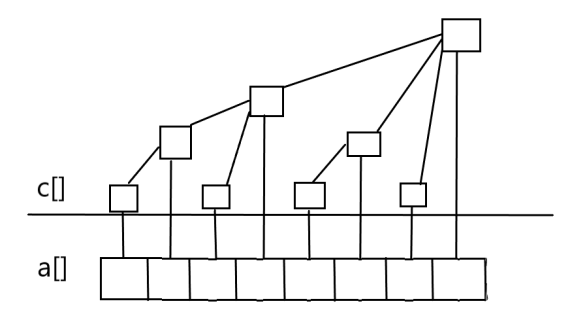如图所示，横线下方为a数组表示为初试数据；上方为数组c，利用树形结构存储a数组内的数据。我们列举出来的这些：

c=a

c=a+a

c=a

c=a+a+a+a

c=a

c=a+a

c=a

c=a+a+a+a+a+a+a+a

......

当我们列举出来这些数据的时候，我们发现：

①所有下标为奇数的c数组都为对应的a数组的值。

②所有下标为2的k次幂的c数组都为对应a数组2的k次幂的前缀和。

③其它下标为偶数的c数组都是由该偶数前所有的值的和，减去该下标对应的最大的2的k次幂的值的和。

即c[i] =a[i - +1] + a[i -+2] + ... + a[i];   //k为i的二进制中从最低位到高位连续零的长度（该偶数的2进制数的最低位1的位置再-1。

当我们计算7的前缀和，即我们需要计算sum=c+c+c;

即我们可以拓展为SUM = C[i] + C[i-] + C[(i - ) - ] + .....；//k1k为i的二进制中从最低位到高位连续零的长度,k2为i-后从最低位到高位连续零的长度。

我们引入lowbit(x)函数，此函数操作为x&(-x)。这里的-x在计算机中是以补码的形式计算的。此函数的作用为：当x为0时，返回为0；当x为奇数时，返回1；当x为偶数时，且为2的m次方时，返回x；当x为偶数，却不为2的m次方的形式时，返回，其中k为二进制中从最低位到高位连续零的长度（该偶数的2进制数的最低位1的位置再-1）。

注意事项：树状数组能够有效的解决单点更新，区间查询的过程。

题目引入：树状数组

代码：

#include<iostream>
#include <stdio.h>
#include<string.h>
using namespace std;
int a;
int c;
int lowbit(int i){
return i&(-i);
}
void update(int i,int x,int n){
while(i<=n){
c[i]+=x;
i+=lowbit(i);
}
}
int downdate(int i){
int sum=0;
while(i>0){
sum+=c[i];
i-=lowbit(i);
}
return sum;
}
int main(){
int n,m;
cin>>n>>m;
for(int i=1;i<=n;i++){
cin>>a[i];
update(i,a[i],n);
}
int x,y,z;
for(int i=1;i<=m;i++){
cin>>x>>y>>z;
if(x==1)update(y,z,n);
else cout<<downdate(z)-downdate(y-1)<<endl;

}
return 0;
}

区间更新，单点查询

我们使用差分数组来表示，即D[i]=a[i]-a[i-1](i>=1);//建议看这里，有比较详细的介绍和说明

区间更新：

例如我们需要更新区间[2,6]，将[2,6]区间内的每一个都加上x，x可正可负；

则：

D=(a+x)-(a)  =a-a+x=D+x

D=(a+x)-(a+x)=a-a  =D

D=(a+x)-(a+x)=a-a  =D

D=(a+x)-(a+x)=a-a  =D

D=(a+x)-(a+x)=a-a  =D

D=(a)  -(a+x)=a-a-x=D-x

即若更新[2,6],将区间[2,6]区间内的每一个都加上x,则就相当于D+x,D-x。

我们推广到一般情况，如果更新区间[a,b],且将[a,b]区间内加上x，则相当于D[a]+x,D[b+1]-x。

单点查询：

若想查询a[n],则根据累加法：

a[n]=D[n]+a[n-1]

a[n-1]=D[n-1]+a[n-2]

...

a=D+a

a=D+a

a=D+a

a=0

这种情况我们则需要用树状数组了。

即用D数组来建立一个树状数组。

区间更新，区间查询

对于求[1,n]区间内的总和sum，我们有如下处理：

已知

=n*(D+D+...+D[n])-(0*D+2*D+...+(n-1)*D[n])

=

这样我们得到了两个，即可用于树状数组。

我们令c数组为(i-1)*D[i]。

所以，我们设置两个树状数组sum1[i]<-D[i]和sum2[i]<-c[i]。

区间更新：

若更新区间[a,b],并且在区间同时+x,对于sum1，我们可以在a处向上更新x，在b+1处更新-x；对于sum2，我们可以在a处向上更新(a-1)*x在b+1处向上更新b*-x;

区间查询：

若查询区间[l,r],我们可以找到区间[0,l-1]的值，向下查找sum1-sum2，找到[0,r]，向下查找sum1-sum2；

代码：

#include<bits/stdc++.h>
int n,m;
int a = {0};
int sum1;    //(D + D + ... + D[n])
int sum2;    //(1*D + 2*D + ... + n*D[n])
int lowbit(int x){
return x&(-x);
}
void updata(int i,int k){
int x = i;    //因为x不变，所以得先保存i值
while(i <= n){
sum1[i] += k;//更新sum1
sum2[i] += k * (x-1);//更新sum2
i += lowbit(i);
}
}
int getsum(int i){        //求前缀和
int res = 0, x = i;
while(i > 0){
res += x * sum1[i] - sum2[i];//这里因为sum1没有乘以n，所以在出结果时成
i -= lowbit(i);
}
return res;
}
int main(){
cin>>n;
for(int i = 1; i <= n; i++){
cin>>a[i];
updata(i,a[i] - a[i-1]);   //输入初值的时候，也相当于更新了值
}
//[x,y]区间内加上k
updata(x,k);    //A[x] - A[x-1]增加k
updata(y+1,-k);  //A[y+1] - A[y]减少k
//求[x,y]区间和
int sum = getsum(y) - getsum(x-1);
return 0;
}

当然，对于区间查询，区间更新的问题，我么还可以使用线段树来处理。

树状数组求逆序对数

我们求逆序对数除了归并算法求，还可以树状数组求逆序对数。

步骤：

• 离散化：这里所谓离散化就是将一个序列的相对大小表示出来。目的是为了方便数据存储。对于重复的数据，我们就依据数据的先后顺序来处理

例如序列：6 25 9 63 2

序列的离散化结果为2 4 3 5 1

例如序列5 60 5 3 2 3

序列的离散化结果为4 6 5 2 1 3

• 树状数组求逆序对数

我们怎样求逆序对数呢？

我们可以依次从后向前遍历，以遍历的时间为序列的顺序，向前查找并且更新。

例如此序列逆序为1 5 3 4 2，则第一次寻找1前面的数据有多少比1小（向下寻找），然后把1向上更新+1；第二次寻找5前面的数据，发现c=1,即5前面有1个比5小的数，然后将5向上更新+1。

题目引入：树状数组求逆序对

代码：

#include<bits/stdc++.h>
using namespace std;
struct node{
long long sum;
long long j;
long long k;
};
long long sum=0;
node a;
long long c;
long long lowbit(long long x){
return x&(-x);
}
bool cmp(const node xx,const node yy){
if(xx.sum==yy.sum&&xx.j<yy.j)return true;
if(xx.sum<yy.sum)return true;
else return false;
}
bool cmp1(const node xx,const node yy){
if(xx.j<yy.j)return true;
else return false;
}
int main(){
long long n;
cin>>n;
for(long long i=1;i<=n;i++){
cin>>a[i].sum;
a[i].j=i;
}
sort(a+1,a+1+n,cmp);
for(long long i=1,x=1;i<=n;i++){
a[i].k=x;
x++;
}
sort(a+1,a+1+n,cmp1);
for(long long i=n;i>=1;i--){
long long x=a[i].k;
long long y=x;
while(y>0){
sum+=c[y];
y-=lowbit(y);
}
while(x<=n){
c[x]++;
x+=lowbit(x);
}
}
cout<<sum;
return 0;
} 

展开全文数据结构
• 洛谷上关于树状数组的一些简单例题，可参考学习，适合初学者
• 树状数组

# 树状数组

树状数组是一个查询和修改复杂度都为log(n)的数据结构。主要用于数组快速单点修改，和快速区间求和.

## 引子

不结合示例分析的题解都是流氓，这里给出leetcode上的一道问题来说明树状数组的特点和优势

简单描述就是，对于一个给定的数组A，希望能够设计一个update函数来修改其中一个数的值，然后再设计一个sum函数来计算数组下标再给定参数l和r之间的值之和。关键点在于这两个函数可能被无数次调用，所以需要保证两个函数的复杂度都要小。

### 暴力算法（不佳）

update函数采用对数组直接修改的方法。

对于sum函数，采用暴力算法。就是对于用例中的每一个l和r，都将它们之间的所有数求和。但是这道题的操作数很大，会超时。

### 前缀和算法（不佳）

sum函数，考虑前缀和的方法，也就是采用动态规划的方法，设计一个新的数组pre来表示前缀和。

其基本思想为，pre[i]表示A+A+A+...+A[i-1]+A[i]。具体操作的时候我们只需要设置pre[i] = pre[i-1]+A[i]即可。

然后如果希望计算数组下标l到r的区间和，只需要计算pre[r]-pre[l-1]即可。希望使用这种方式达到简化计算复杂度的目的。

但是这时候考虑update函数，当我们对数组A直接进行修改值之后，我们发现pre数组也需要对应进行修改，下面通过示例进行说明：

原数组：A= {1,2,3,4,5}

前缀和数组：pre = {1,3,6,10,15}

分析：当我们对A修改，将其原值3改为2的时候。

考虑到pre数组是由A数组得到的,因为

pre = A

pre = A+A

pre = A+A+A

pre = A+A+A+A

pre = A+A+A+A+A

我们会发现，A的修改会影响到pre和pre，所以我们需要对pre和pre都进行修改

pre新值 = A+A+A+A新值

pre新值 = A+A+A+A新值+A

综上，归纳可以得知，如果修改了A[i]的值，对于所有j>=i, pre[j]的值都需要改变，具体改变量为pre[j] = pre[j] + A[i]新值 -A[i]原值

这样一来，对于update函数，我们的复杂度就会提高，可以认为是O(n)，即使当前sum函数复杂度变为了O(1)，这道题总的复杂度依然是O(1)。

### 痛点

针对上述问题我们发现，需要做到两点：

首先需要能够快速计算区间和，其次要保证在修改了数组的值之后，对相关数据结构内容修改的操作数也要尽量少

这里总结上述分析，我们知道，希望能够设计新的数据结构，让它能够同时包含多个节点信息，类似pre数组，这样计算区间和就能够提速。然后希望这个数据结构的更新也要尽量快。这里就引入了树状数组的概念。

## 树状数组

二叉树大家一定都知道，如下图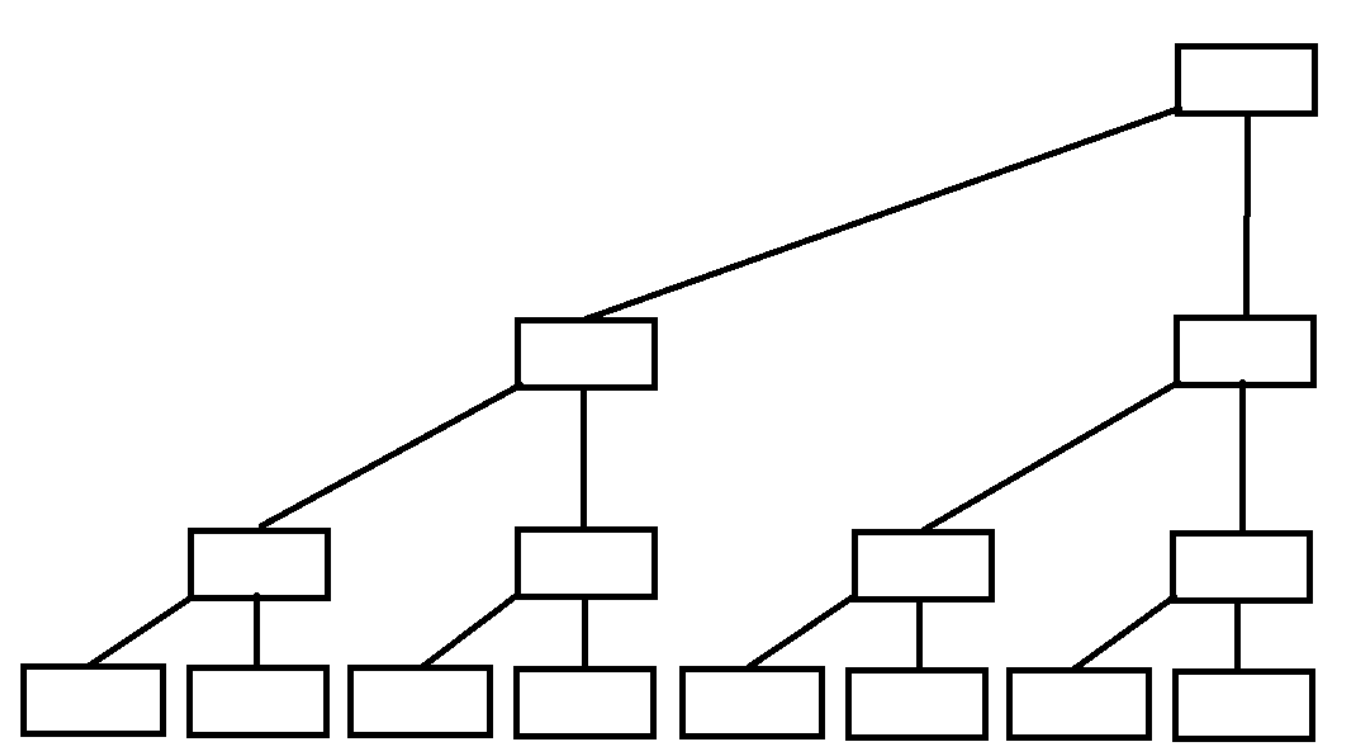如果每个父亲都存的是两个儿子的值，是不是就可以解决这类区间问题了呢。是的没错，但是这样的树形结构，叫做线段树。

那真的的树形结构是怎样的，和上图类似，但省去了一些节点，以达到用数组建树。

对于一般的二叉树,我们是这样画的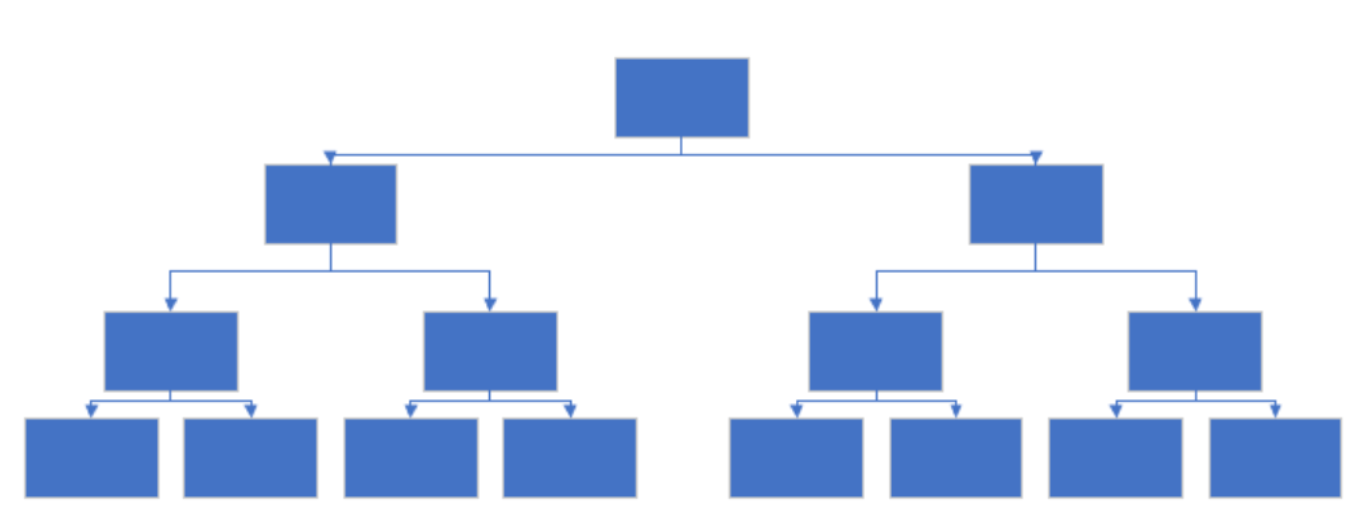把位置稍微移动一下,便是树状数组的画法，最下面一行为原数组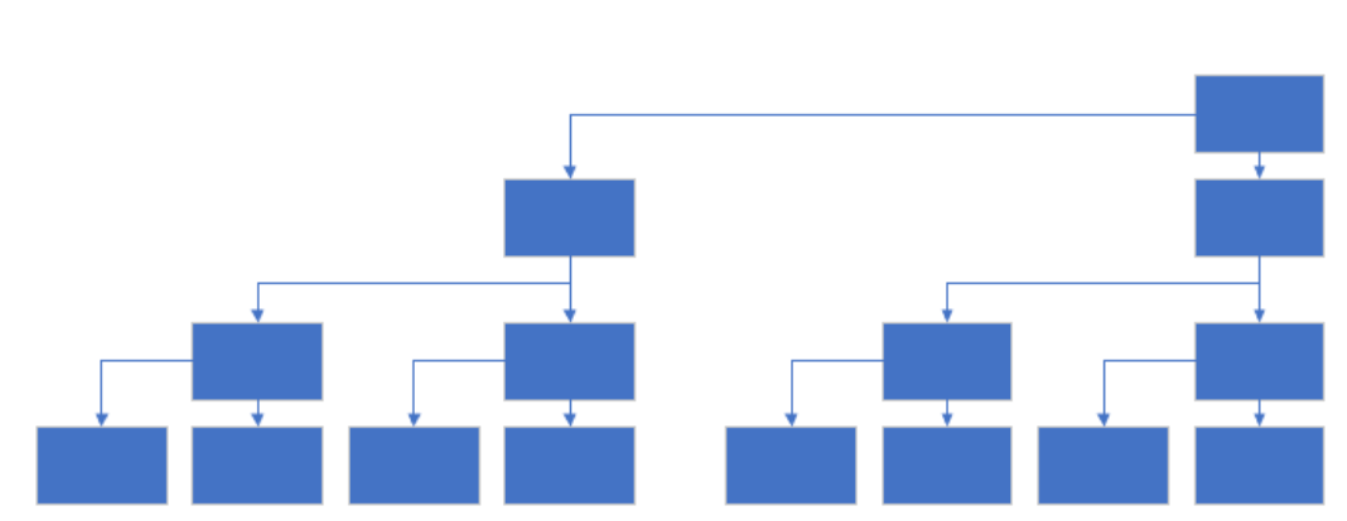需要注意的是，图中的子节点包括自己，比如说8这个节点，里面的值是原始数组中[5，6，7，8]的和

这里首先我们将原数组称为A数组，树状数组称为C数组

需要设计两个过程，更新过程和查询过程

这两个过程就是树状数组的最核心应用，对应快速修改节点值，快速计算前缀和两个功能

参考下图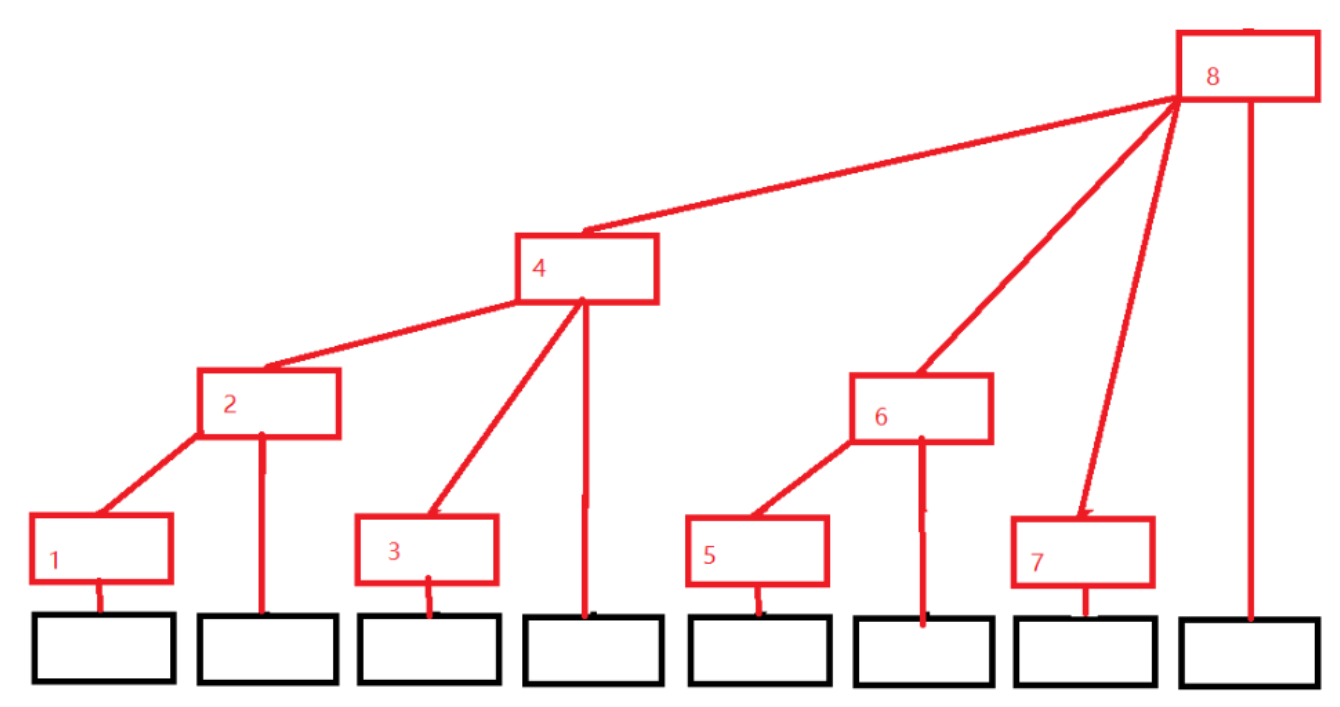最底下一行黑色节点从左到右为原数组A,而带有数字的红色节点就是树状数组C的节点

我们可以看到比如说C8节点，它的子节点有C4，C6，C7，A8(第八个黑色节点)而C4，C6，C7由分别有自己的子节点。

根据这张图，对于8节点可以表示成：

C = C + C +C +A

换一张图来理解一下：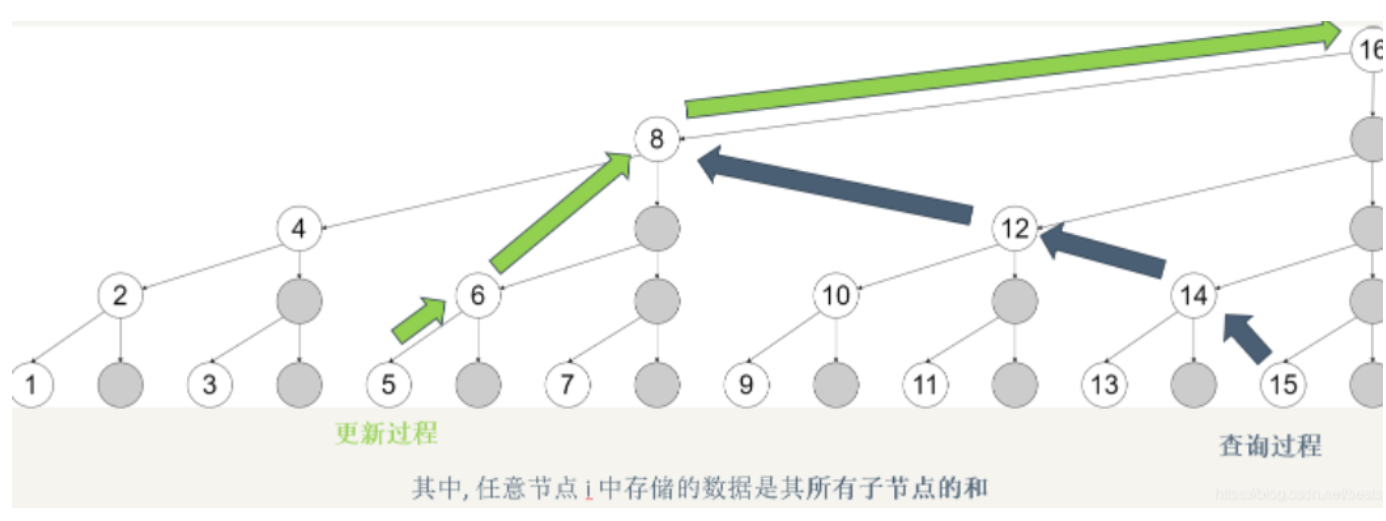这张图里面表示的所有数字节点就是树状数组C，最下面一行从左到右同时也表示原数组A（包括灰色节点）。图中标出了修改节点5需要修改的所有节点值的更新过程和计算下标0~15的节点值之和的查询过程

### 更新过程

根据上面树状数组的计算，我们参考这张图能够知道，C5是C6的子节点，而C6是C8的子节点，当我们修改A5的时候

因为C5 = A5，所以C5会变化，导致C6变化，再导致C8变化，那么变化的值为多少呢，均为 A5新值减去A5旧值

这里的思想和之前提到的前缀和有点相似，前缀和中是将pre5及后面所有的节点都要修改(因为这些节点都包含了5节点的信息)，但是这里只需要修改C5，C6和C8节点的信息（因为只有这几个节点包含了5节点的信息），总体来说比起前缀和方法更新节点的时候对数据结构的更新就很快了

下面有人会问，你这是画图看出来要更新C5，C6和C8。那么具体是修改了哪些节点呢？这里我们要从二进制的角度来看，重新画一张二进制的图，将所有节点上的十进制改为了二进制表示：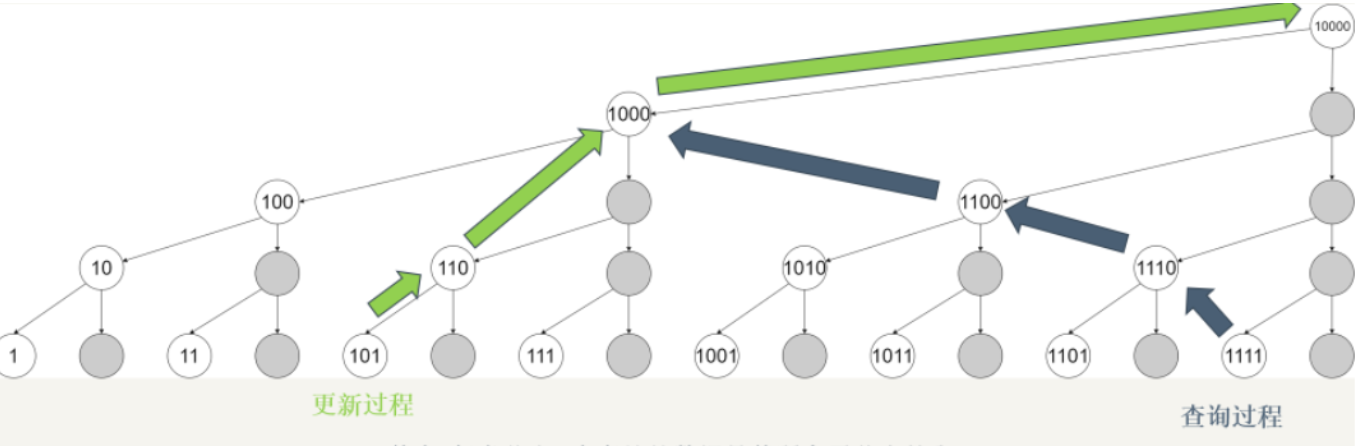对应过来，我们会发现C101的值变化后，会影响C110，进而影响C1000的值

那么101和110和1000之间有什么关系呢？

可以归纳得出，110是由101加上1得到，1000是由110加上10得到

101加1是因为101中只保留最低位的1就是1， 而110加10是因为110中只保留最低位1是10

所以我们只需要算出当前节点下标二进制表示的最低位1的位置，然后算出只保留这个1得到的数，结合当前节点的下标，就可以计算出下一个需要修改的节点的下标了

这里我们设计一个函数lowbit(int x)用于计算给定一个下标x，返回：其二进制下标只保留最低位1会得到的那个数

//借鉴前人智慧，可以轻松得到
int lowbit(x){return x&(-x);}

简单解释一下为什么可以通过x&(-x)得到这个数

我们知道,对于一个数的负数就等于对这个数取反+1

以二进制数11010为例:11010的补码为00101,加1后为00110,两者相与便是最低位的1

其实很好理解,补码和原码必然相反,所以原码有0的部位补码全是1,补码再+1之后由于进位那么最末尾的1和原码

最右边的1一定是同一个位置(当遇到第一个1的时候补码此位为0,由于前面会进一位,所以此位会变为1)

所以我们只需要进行a&(-a)就可以取出最低位的1了

//tr表示树状节点
/**
* 用于进行树状数组的更新过程
* @param x: 被修改的数组下标
* @param val: 修改量（负数代表减少）
*/
public void add(int x,int val){
for(int i=x;i<=n;i+=lowbit(i)){
tr[i] += val;
}
}

整个复杂度为O(logn),因为每次lowbit导致移了一位，相当于反复对数组长度n除以2直到0的复杂度。

### 查询过程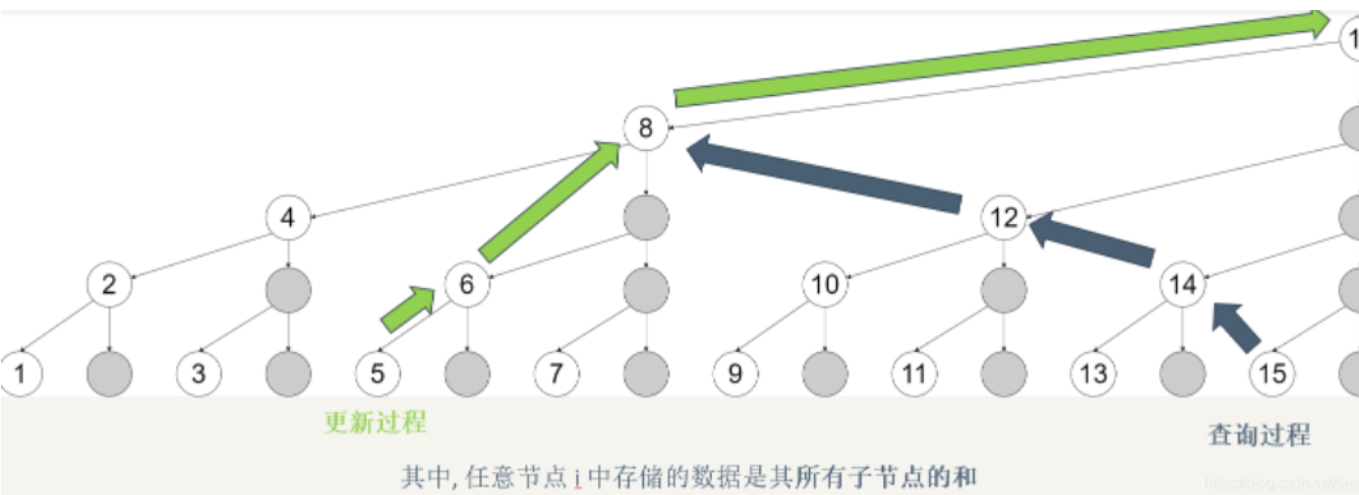查询过程是计算从A到A[i]的所有值进行求和

如果我需要查询A~A之和,观察当前图我们会发现

8节点包含了1~8，12节点包含了9~12，14节点包含了13~14，15节点包含了15

那我们只需要将C8,C12,C14,C15进行求和即可

但是问题又来了，这是画图发现的，具体操作的时候需要对哪几个C进行求和呢？

同样的我们将其转换为二进制表示的图：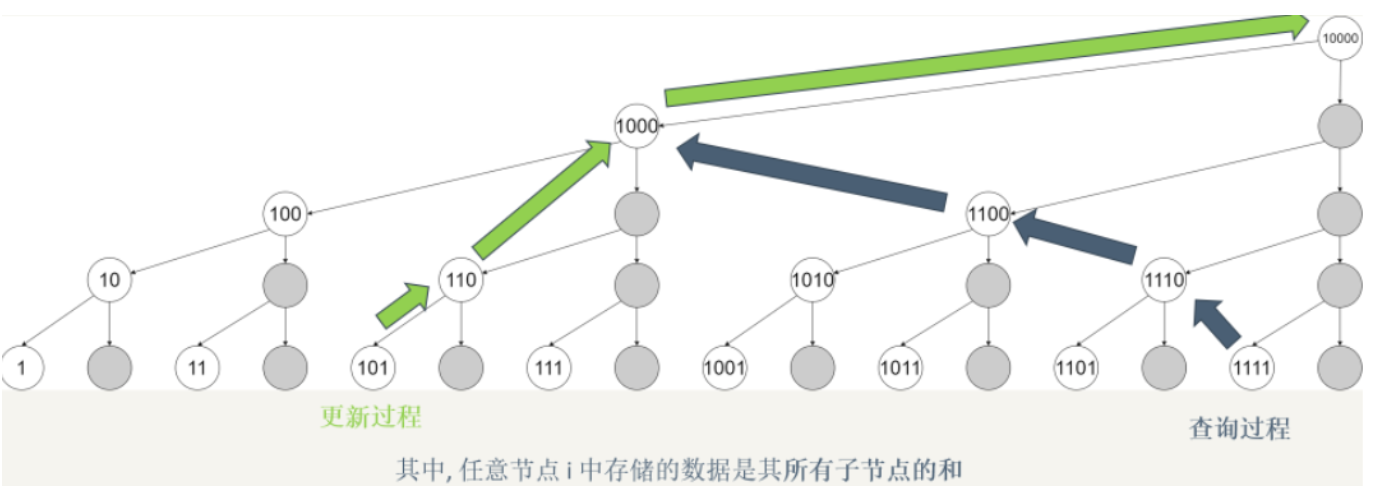首先对sum置零。

归纳可得，对于15的二进制节点1111，我们减去1111保留最低位1的数1，得到1110.然后让sum加上节点1110的值。

然后对于1110，同样减去其保留最低位1的数10得到1100，然后让sum加上节点1100的值。

再对于1100，减去其保留最低位1的数100得到1000，然后让sum加上节点1000的值。

再对于1000，减去其保留最低位1的数1000得到0。这时不能再算了，因为已经到头了。

所以我们可以发现，又需要用到lowbit这个函数了。基于上述思想，整个计算前缀和流程可以表示如下：

//tr表示树状节点
/**
* 用于进行前缀求和的查询过程
* @param x: 需要查询的数组下标x
* @return 计算从0节点到x节点之间所有值之和
*/
public int pre_sum(int x){
int sum = 0;
for(int i=x;i>0;i-=lowbit(i)){
sum += tr[i];
}
return sum;
}

查询过程相当于是求前缀和的过程，那么有了前缀和，我们就可以通过前缀和的作差得到其中部分区间和的答案了。

这里使用到的复杂度为O(logn)，因为每次lowbit移了一位，相当于反复对数组长度n除以2直到0的复杂度。

## 代码参考

至此，所有的细节就完成了，下面给出针对leetcode上问题的具体题解

307. 区域和检索 - 数组可修改 - 力扣（LeetCode） (leetcode-cn.com)

class NumArray {
private int[] tr;
private int n;
private int[] nums;
public NumArray(int[] nums) {
this.n = nums.length;
this.tr = new int[n+1]; //注意，下标0的值设置为默认的0，是为了处理的方便
this.nums = nums;
for(int i=0;i<n;i++){
}
}

public void update(int index, int val) {
this.nums[index] = val;
}

public int sumRange(int left, int right) {
return pre_sum(right+1)-pre_sum(left);
}
​
public int lowbit(int x){
return x&(-x);
}
​
public int pre_sum(int x){
int sum = 0;
for(int i=x;i>0;i-=lowbit(i)){
sum += tr[i];
}
return sum;
}
​
public void add(int x,int val){
for(int i=x;i<=n;i+=lowbit(i)){
tr[i] += val;
}
}
}

## 模板

这里参考了三叶姐的树状数组的模板,还请大家多多去给她捧场

class NumArray {
int[] tree;
int lowbit(int x) {
return x & -x;
}
int query(int x) {
int ans = 0;
for (int i = x; i > 0; i -= lowbit(i)) ans += tree[i];
return ans;
}
void add(int x, int u) {
for (int i = x; i <= n; i += lowbit(i)) tree[i] += u;
}
int[] nums;
int n;
public NumArray(int[] _nums) {
nums = _nums;
n = nums.length;
tree = new int[n + 1];
for (int i = 0; i < n; i++) add(i + 1, nums[i]);
}
public void update(int i, int val) {
add(i + 1, val - nums[i]);
nums[i] = val;
}
public int sumRange(int l, int r) {
return query(r + 1) - query(l);
}
}

## 参考博客

(72条消息) 树状数组 数据结构详解与模板(可能是最详细的了)bestsort的博客-CSDN博客树状数组

我喜欢你啊。 (cnblogs.com)

展开全文leetcode java
• 树状数组是一个查询和修改复杂度都为log(n)的数据结构。主要用于查询任意两位之间的所有元素之和，但是每次只能修改一个元素的值；经过简单修改可以在log(n)的复杂度下进行范围修改，但是这时只能查询其中一个元素的...数据结构
• 用java实现的树状数组，可以作为一个简单的模版来进行应用，如果有不懂得地方，可以上网查找树状数组的原理java 树状数组
• 数据结构之树状数组 目录 数据结构之树状数组 1:引子~~~ 假如现在需要你维护一种数据结构，需要能支持区间查询和单点更新，那么该怎么办？ 方法一：暴力枚举 不用想，TLE妥妥的 ...树状数组
• } // 递归遍历 function traverseTree(node){ if (!node) { return; } traverseNode(node); if (node.children && node.children.length > 0) { var i = 0; for (i = 0; i ; i++) { this.traverseTree(node....
• 对于一个新入门前端的人来说，一切的数据都是基本后端处理完成，但是遇到只处理sql的后端，那么一些数据的操作就要弄到前端，那么如何对一个数组对象，合并为一个完整的树形数组1.使用foreach循环 2.使用filter...
•大数据
• 本文详细介绍了树状数组的原理，并用树状数组解校门外的这个问题.
• 数据结构 树状数组套主席 结构详解 ???? | Powered By HeartFireY 文章目录数据结构 树状数组套主席 结构详解一、简述二、详解1.结构理解2.区间修改3.区间第kkk大查询三、板子 一、简述 主席主要用来解决静态...数据结构 树结构 acm竞赛
• 在生成多级树状数组之前，我们一般得到的数据结构如下[{"id": 6,"parent_id": 5,"name": "体育专题1","intro": "体育专题1111介绍",}, {"id": 5,"parent_id": 4,"name": "体育专题","intro": "体育专题介绍",}, {"id...php 生成树形数组
• 通过了解树状数组的原理和定义来解决有关给定一个由n个不同的整数组成的序列，最少需要交换多少次交换相邻的两个数，使其升序排列。
• JS–数组树状数组排列 2020年9月10日 代码 function getArrayTree(arrList, id, fid, children = 'children') { let map = [] arrList.forEach(item => { let up = arrList.filter(x => x[id] == item...
• 因为子树树形被破坏，在做减法时，子节点子树对父节点的贡献不能用子树维护的信息 + 连向父节点的边的贡献得到，考虑在每个节点再维护一个线段树，按距离建树用来统计子树内的节点到其父节点距离为 p 的点权和，这样...
• 【BZOJ3196】二逼平衡树状数组，线段） [BZOJ3196]二逼平衡(树状数组,线段) 题面 BZOJ题面 题解 如果不存在区间修改操作: 搞一个权值线段 区间第K大--->直接在线段树上二分 某个数第几大--->查询一下 ....
• 见名知意，就是用数组来模拟树形结构。那么又衍生出一个问题，为什么不直接建树呢？这个问题的答案是没有必要，树状数组就可以解决的问题没必要建树。 2. 用来解决什么问题？ 和线段树一样用来解决区间上的更新、...
• 树状数组，又称二进制索引树状数组的经典实现包含两个数组：一个是存储数列元素的数组 A[]，另一个是存储数列前缀和的数组 C[]。而树状数组名称的由来，恰是因为数组 C[] 呈现为树状结构。两个数组之间的关系为...树状数组
• 树状数组：顾名思义，用数组来模拟树形结构。 树状数组可以解决的问题：区间上的更新以及求和问题。以**o(logn)**获得任意（区间）前缀和 树状数组可以解决的问题都可以用线段树解决，那么区别在于，树状数组的系数...leetcode 排序算法
• ## 树状数组（简单介绍）

万次阅读 多人点赞 2020-02-20 12:03:06
树状数组解决的问题： 假如有这样一种情景，先输入一个长度为n的数组，然后我们有如下两种操作： 输入一个数m，输出数组中下标1~m的前缀和 对某个指定下标的数进行值的修改 多次执行上述两种操作； 常规方法 对于...
• ## 树状数组 算法详解

千次阅读 2018-09-28 20:36:11
这几天扫描知识点，扫描到了树状数组就解决了。 首先什么是树状数组？它其实就是支持单点修改和区间查询的数据结构，我会一步一步讲解这个树状数组到底是个什么东西，所以请跟上我的步骤，拿出纸笔跟我一起计算。 ...树状数组
• 当我们需要用到数组来存放数据并对数据进行操作时，往往有这么几种数组形式： 1.普通数组： 修改操作：令 a[x]+=k ，时间复杂度O(1) 询问操作：输出a[x]+a[x+1]+a[x+2]+…+a[y-1]+a[y] ，时间复杂度O(n) 2....
• 我们了解了一维树状数组的原理，二维树状数组和一维树状数组类似，在二维树状数组中，arr[x][y]arr[x][y]arr[x][y]记录的是右下角为​(x,y)​(x,y)​(x,y)，高度为lowbit(x)lowbit(x)lowbit(x)，宽度为lowbit(y)...数据结构...